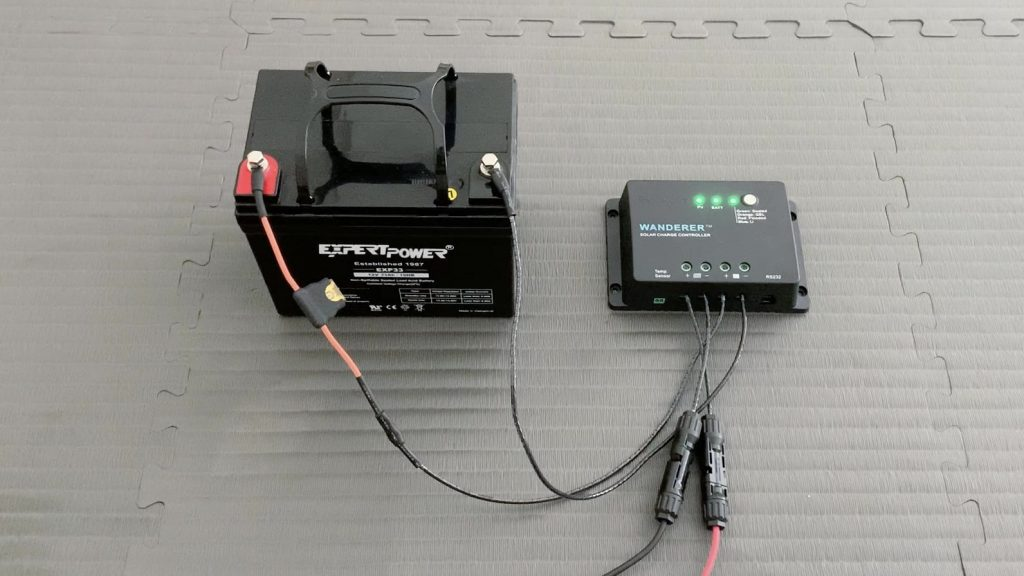# Watt Hours to Amp Hours (Wh to Ah) Conversion Calculator

## Watt Hours to Amp Hours Conversion Calculator

Error: Enter a valid number of watt hours (Wh).
Error: Enter a valid voltage (V).
amp hours (Ah)
Conversion formula: Ah = Wh / V

## Watt Hours to Amp Hours Conversion Chart

Here's a conversion chart converting common watt hour values to amp hours at 12 and 24 volts.

## How to Convert Watt Hours to Amp Hours (Wh to Ah)

To convert watt hours to amp hours, simply divide watt hours by volts:

`Amp hours (Ah) = Watt hours (Wh) ÷ Volts (V)`

### Example

Let's say you have a 2000Wh LiFePO4 battery. You know it's a 12 volt battery, and you want to calculate its amp hours.

To do so, you'd divide watt hours by volts:

`2000Wh ÷ 12V = 166.67Ah`

In this example, your battery has a 166.67Ah capacity.

Note: Most people use a battery's nominal voltage when calculating amp hours or watt hours, but it's worth reminding you that a battery's actual voltage is slightly different and varies based on its remaining capacity. For instance, a 12V LiFePO4 battery actually has a voltage of 13.6V when fully charged.

### Why Convert Watt Hours to Amp Hours?

Some brands have started reporting their batteries' capacities in watt hours -- particularly for solar generators (aka portable power stations).

This is helpful for making an apples to apples comparison of the energy these batteries can deliver.

But, it can be helpful to convert watt hours to amp hours when designing a solar electrical system. After all, things like wire gauge, fuse size, and max charge and discharge rates depend on amperage, not wattage.Why convert watt hours to amp hours? One reason is because it can help you figure out the max charge rate of your battery, so you can design a solar power system that doesn't go over that limit.

For example:

The LiFePO4 battery in the example above had a 166.67Ah capacity. This is important to know because many LiFePO4 batteries have a maximum recommended charge rate of 50% of battery capacity in amp hours.

This means our battery's max charge rate is 83.3 amps, and we can design our solar power system so we don't exceed that limit.

## How to Convert Amp Hours to Watt Hours (Ah to Wh)

To convert amp hours to watt hours, you just multiply amp hours by volts:

`Watt hours (Wh) = Amp hours (Ah) × Volts (V)`

Calculator: Amp Hours to Watt Hours Calculator

### Example

Let's say you have a 100Ah 12V car battery.

To calculate your battery's watt hours, you'd multiply 100Ah × 12V:

`100 amp hours (Ah) × 12 volts (V) = 1200 watt hours (Wh)`

In this example, your car battery has a capacity of 1200Wh.

## How to Convert Kilowatt Hours to Amp Hours (kWh to Ah)

1 kilowatt hour is equal to 1000 watt hours. (Just like how 1 kilometer is 1000 meters.)

So, to convert kilowatt hours to amp hours, you multiply kilowatt hours by 1000 and then divide by volts.

`Amp hours (Ah) = Kilowatt hours (kWh) × 1000 ÷ Volts (V)`

### Example

Let's say your buddy tells you he built a 3.5kWh 24V solar generator. Impressive. You wonder: How many amp hours is his generator's battery?

To find out, you'd do the following:

`3.5kWh × 1000 ÷ 24V = 145.83Ah`

Turns out his generator's battery has a capacity of around 145Ah. Time for you to build one with a 150Ah capacity. 😉

## How to Convert Watt Hours to Milliamp Hours (Wh to mAh)

1 amp hour is equal to 1000 milliamp hours. (Just like how 1 meter is 1000 millimeters.)

So, to convert watt hours to milliamp hours, you multiply watt hours by 1000 and then divide by volts.

`Milliamp hours (mAh) = Watt hours (Wh) × 1000 ÷ Volts (V)`

### Example

Let's say you're interested in buying a new phone with a 12.4 watt hour battery. You know it's a 3.7 volt lithium ion battery and you want to find out how many milliamp hours it is.

To calculate its capacity in milliamp hours, you'd do the following:

`12.4Wh × 1000 ÷ 3.7V = 3351.35mAh`

The phone battery has a capacity of around 3351mAh.

## More Solar Calculators